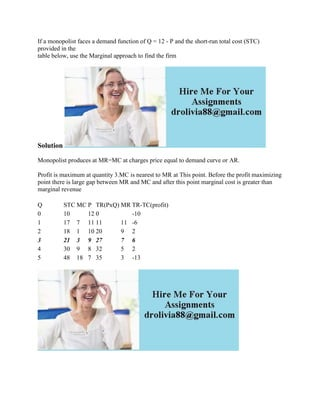Diese Präsentation wurde erfolgreich gemeldet.

# If a monopolist faces a demand function of Q - 12 - P and the short-ru.docx

Anzeige
Anzeige
Anzeige
Anzeige
Anzeige
Anzeige
Anzeige
Anzeige
Anzeige
Anzeige
Anzeige×

1 von 1 Anzeige

# If a monopolist faces a demand function of Q - 12 - P and the short-ru.docx

If a monopolist faces a demand function of Q = 12 - P and the short-run total cost (STC) provided in the
table below, use the Marginal approach to find the firm
Solution
Monopolist produces at MR=MC at charges price equal to demand curve or AR.
Profit is maximum at quantity 3.MC is nearest to MR at This point. Before the profit maximizing point there is large gap between MR and MC and after this point marginal cost is greater than marginal revenue

.

If a monopolist faces a demand function of Q = 12 - P and the short-run total cost (STC) provided in the
table below, use the Marginal approach to find the firm
Solution
Monopolist produces at MR=MC at charges price equal to demand curve or AR.
Profit is maximum at quantity 3.MC is nearest to MR at This point. Before the profit maximizing point there is large gap between MR and MC and after this point marginal cost is greater than marginal revenue

.

Anzeige
Anzeige

### If a monopolist faces a demand function of Q - 12 - P and the short-ru.docx

1. 1. If a monopolist faces a demand function of Q = 12 - P and the short-run total cost (STC) provided in the table below, use the Marginal approach to find the firm Solution Monopolist produces at MR=MC at charges price equal to demand curve or AR. Profit is maximum at quantity 3.MC is nearest to MR at This point. Before the profit maximizing point there is large gap between MR and MC and after this point marginal cost is greater than marginal revenue Q STC MC P TR(PxQ) MR TR-TC(profit) 0 10 12 0 -10 1 17 7 11 11 11 -6 2 18 1 10 20 9 2 3 21 3 9 27 7 6 4 30 9 8 32 5 2 5 48 18 7 35 3 -13